##### Print Instructions

NOTE: Only your test content will print.
To preview this test, click on the File menu and select Print Preview.

See our guide on How To Change Browser Print Settings to customize headers and footers before printing.

# Functions in Excel (Grade 10)

Print Test (Only the test content will print)

## Functions in Excel

1.
Describe the task that is performed by the button circled in the image below.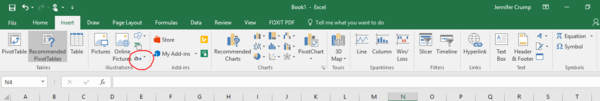2.
Describe the task that is performed by the button circled in red in the below.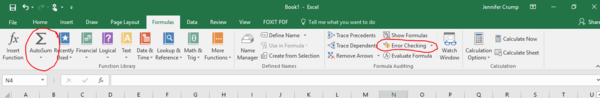3.
Describe the task that is performed by the button circled in red in the image below.4.
Describe the task that is performed by the button circled in red in each of the images below.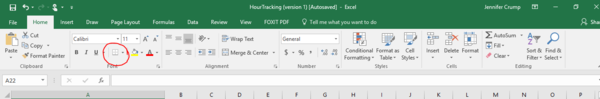5.
Describe the task that is performed by the button circled in red in each of the images below.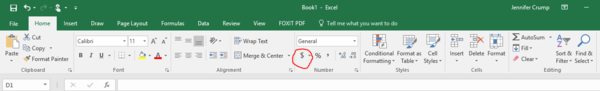6.
Describe the task that is performed by the button circled in red in each of the images below.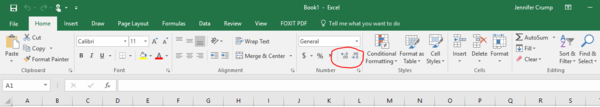7.
Describe the task that is performed by the button circled in red in each of the images below.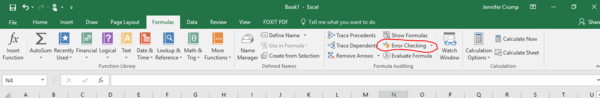8.
Describe the task that is performed by the button circled in red in the image below.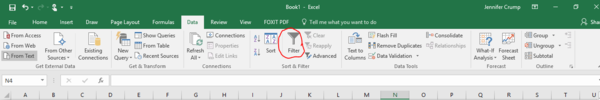9.
Describe the task that is performed by the button circled in red in each of the images below.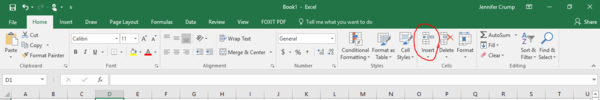10.
Describe the task that is performed by the button circled in red in the below.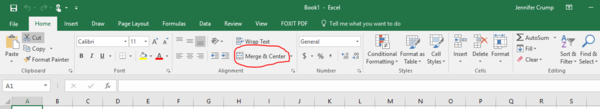11.
Describe the task that is performed by the button circled in red in each of the images below.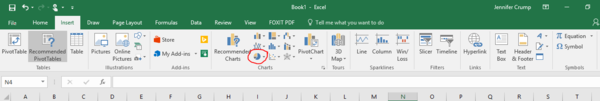12.
Describe the task that is performed by the button circled in red in each of the images below.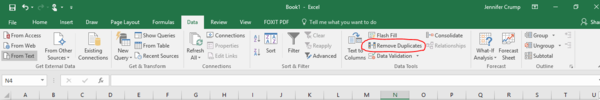13.
Describe the task that is performed by the button circled in red in each of the images below.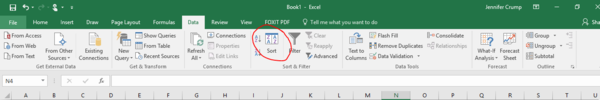14.
Describe the task that is performed by the button circled in red in each of the images below.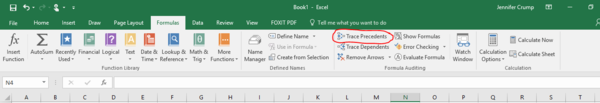15.
Describe the task that is performed by the button circled in red in the image below.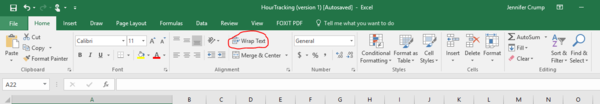You need to be a HelpTeaching.com member to access free printables.
Already a member? Log in for access.    |    Go Back To Previous Page